## Loren on the Art of MATLABTurn ideas into MATLAB

Note

Loren on the Art of MATLAB has been retired and will not be updated.

# MATLAB and Mind Reading Card Games

R2020a is upon us! Do you read the release notes? Today's guest blogger, Toshi Takeuchi, apparently does, and he would like to share some new tricks using one of the new features. He also discusses sharing your code as a MATLAB app, since it is not easy to collaborate with people directly these days.

### Contents

#### UTF-8 Support in MATLAB

As of R2020a, MATLAB defaults to saving new plain text files using UTF-8. What's the big deal, you say? Das ist eine große Sache, それは大ごとだ, because you can now use international characters mixed within English easily. Even if you only speak English, you sure do use Greek letters like π, σ, or β, right? This also means you can use emojis, too, but I can't show it here because publish to html feature doesn't translate emojis into html entities like &#128513;

Personally, however, I am more excited with the playing card symbols. Let's make a deck of 52 cards.

deck = ["♠","♥","♦","♣"] + ["A"; (2:10)';'J';'Q';'K']

deck =
13×4 string array
"♠A"     "♥A"     "♦A"     "♣A"
"♠2"     "♥2"     "♦2"     "♣2"
"♠3"     "♥3"     "♦3"     "♣3"
"♠4"     "♥4"     "♦4"     "♣4"
"♠5"     "♥5"     "♦5"     "♣5"
"♠6"     "♥6"     "♦6"     "♣6"
"♠7"     "♥7"     "♦7"     "♣7"
"♠8"     "♥8"     "♦8"     "♣8"
"♠9"     "♥9"     "♦9"     "♣9"
"♠10"    "♥10"    "♦10"    "♣10"
"♠J"     "♥J"     "♦J"     "♣J"
"♠Q"     "♥Q"     "♦Q"     "♣Q"
"♠K"     "♥K"     "♦K"     "♣K"


That means we can also use those special characters in the plot as well.

figure
rectangle("Position",[1 0.5 1 2],'Curvature',0.2)
text(1.5,1.5,deck(1),"FontSize",36,"HorizontalAlignment","center")
axis([0 3 0 3])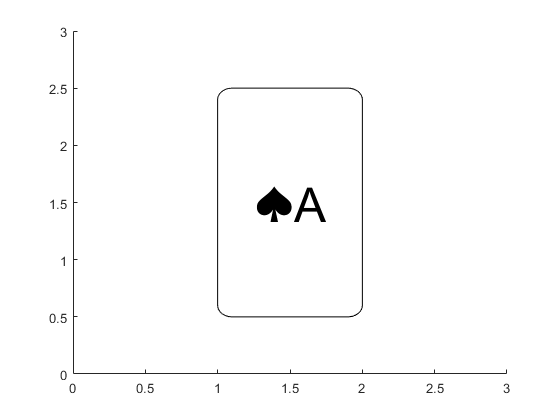#### Mind Reading Card Magic

Let's play the famous 21-card mind reading trick with those cards.

You are entertaining your friends with a card trick. First, shuffle the deck and select first 21 cards from the deck.

rng("default") % for repeatability
picked = initialize21Cards(deck);


Ask a volunteer to choose a card from the stack of 21 cards and remember it, but not tell you what it is.

% select a card
chosenCard = picked(randi(21))

chosenCard =
"♣8"


Have your volunteer shuffle the deck of 21 cards and return it to you.

stacked = picked(randperm(21));


There are several variations to the steps depending on the effect you want to achieve. Here you will lay out the cards on the table. When you do so, you lay them out into 3 columns of 7 cards. There is a reason to do it this way when you are handling physical cards, but let's use a 3x7 layout to make it easier to plot the cards. We substitute columns with rows.

plotCardsFaceUp(reshape(stacked,[3 7]),0)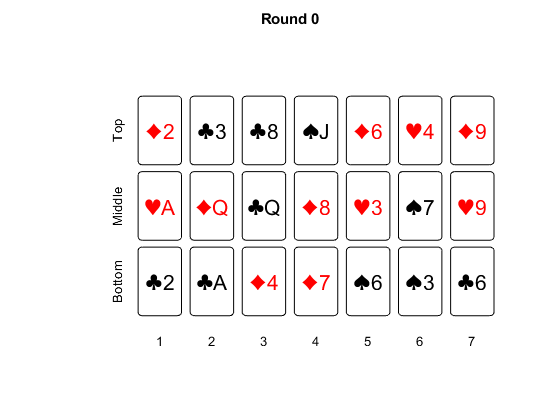Repeat the process 4 times:

1. Deal the cards face up into three rows moving from bottom to top. You should have seven cards in each row.
2. Ask your volunteer to point to the row the chosen card is in: the bottom row, the middle row or the top row.
3. Slide each row of cards together to form three piles, preserving the order.
4. Collect the three piles into one stack in a specific order—make sure that the pile your volunteer pointed to is always collected second.
for ii = 1:4
placed = reshape(stacked,[3 7]); % deal the cards face up
selectedRow = find(any(placed == chosenCard,2)); % row with the card
stacked = restack(placed,selectedRow); % gather cards into a stack
end


Reveal the 11th card in the stack, which should be the chosen card. Hopefully your friends are impressed.

answer = stacked(11);

correctCard =
logical
1


#### How Does It Work?

Spoiler Alert! The trick is revealed!

Now let's see why this works. We will keep it simple by using the first 7 cards from the suits of ♠, ♥ and ♦. We will choose ♦7 for this example.

picked = ["♠","♥","♦"]' + ["A",(2:7)];
chosenCard = "♦7";


Round # 1

When you first lay out the cards, you have no idea where the chosen card is. It could be anywhere. Then your volunteer tells you it is in the top rows. You now know that the chosen card is one of those 7 cards. Let's highlight them.

plotCardsFaceUp(picked,1,"♦" + ["A" (2:7)])Now we collect the cards into a stack in a specific order.

stacked = restack(picked,3);


Because you preserve the order of the respective rows when turning them into piles, and you insert the pile that contains that chosen card in the middle, that card will be placed somewhere between 8th to 14th position.

stacked(8:14)

ans =
1×7 string array
"♦A"    "♦2"    "♦3"    "♦4"    "♦5"    "♦6"    "♦7"


Round #2

Then you deal the stack into 3 rows again. The cards that used to be ordered along the rows now go column by column. The card is now somewhere in the third through fifth position in the row that contains it. Your volunteer tells you it is in the middle row. Now that reduces the possibilities to 3 cards.

placed = reshape(stacked,[3 7]);
plotCardsFaceUp(placed,"♦" + ["A" [4,7]],2)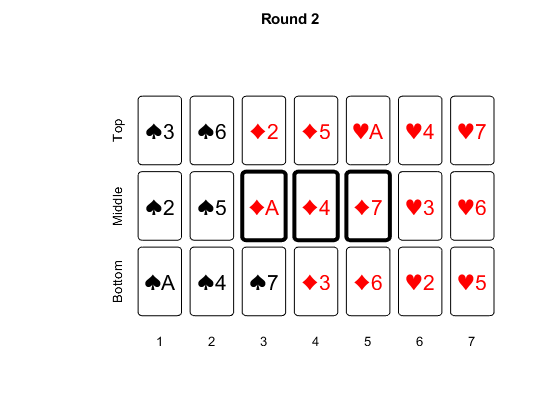You gather the rows into a single stack again as before.

stacked = restack(placed,2);


This ensures that the card will be in the 10th through 12th position of the stack.

stacked(10:12)

ans =
1×3 string array
"♦A"    "♦4"    "♦7"


Round #3

You lay the cards again. This moves the card in the 4th position in one of the rows. Your volunteer tells you it is in the top row. At this point you know that ♦7 is the chosen one.

placed = reshape(stacked,[3 7]);
plotCardsFaceUp(placed,"♦7",3)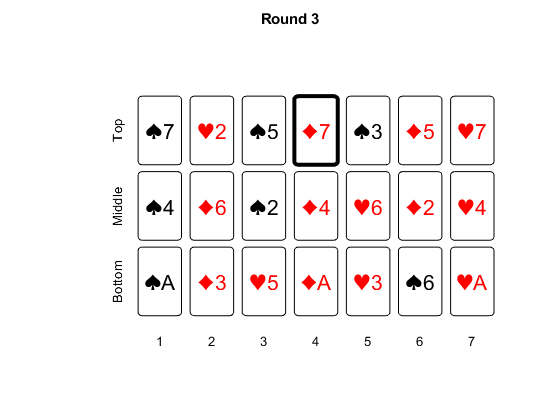You gather the rows into a single deck again.

stacked = restack(placed,3);


Round #4

When you lay the card down, you see that the card moved to the 4th position in the middle row.

placed = reshape(stacked,[3 7]);
answer = placed(2,4); % the 4th position in the middle row

correctCard =
logical
1


At this point you could just point the card, but your volunteer will notice that the card always ends up in the middle row. It is better to collect the cards into a single deck as before and pick the 11th card from the top.

To make it more mysterious, you probably don't want to lay the cards on the table at all. Instead, we can separate the deck into 3 piles of 7 cards and ask your volunteer to peek through them and tell you which pile contains the chosen card. This way, your volunteer will have no visual cue what's going on.

#### Building and Sharing a MATLAB App

Now that we worked out this card trick in code, we might as well turn it into a MATLAB app, right? If you are interested, follow the instructions here and get the app code to build your own app.

Once you build an app, of course you want to share it.

• If your friends are also MATLAB users, you can simply share the .mlapp file, but they will probably apprediate it if you package it so that it comes with the installer.
• How about your friends who doesn't have MATLAB? You can turn this into a standalone desktop app if you use MATLAB Compiler.
• You can also turn it into a web app that runs on browser and make it available over the web hosted on MATLAB Web App Server. See this video for more details.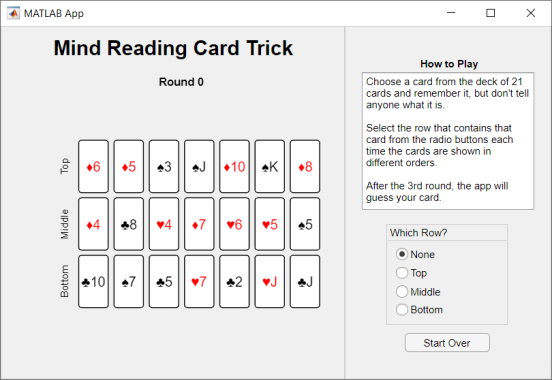#### Summary

Now that you know you can use playing cards in MATLAB, you can try all sorts of algorithms and card tricks and come up with your own apps. Please share your creations here!

#### Local Functions

function picked = initialize21Cards(deck)
shuffledDeck = deck(randperm(numel(deck)));
picked = shuffledDeck(1:21);
picked = picked(randperm(21));
end

function stacked = restack(placed,selectedRow)
rows = randperm(3);
rows = setdiff(rows,selectedRow);
rows = [rows(1), selectedRow, rows(2)];
stacked = [placed(rows(1),:),placed(rows(2),:),placed(rows(3),:)];
end

function plotCardsFaceUp(cards,varargin)
if nargin > 1
for ii = 1:length(varargin)
if isstring(varargin{ii})
highlight = varargin{ii};
else
numRound = varargin{ii};
end
end
end

[recPos,txtPos,seq,axisLim] = positionCards(cards);

if exist("numRound","var") && exist("highlight","var")
plotCards(recPos,txtPos,seq,axisLim,numRound,highlight)
elseif exist("numRound","var")
plotCards(recPos,txtPos,seq,axisLim,numRound)
elseif exist("highlight","var")
plotCards(recPos,txtPos,seq,axisLim,highlight)
else
plotCards(recPos,txtPos,seq,axisLim)
end
end

function [recPos,txtPos,seq,axisLim] = positionCards(cards)
[n,m] = size(cards);
recPos = zeros(n*m,4);
txtPos = zeros(n*m,2);
seq = [];
for ii = 1:n
seq = [seq cards(ii,1:m)];
end

origin = [1.5 1];
w = 1;
h = 2;
spacing = 0.2;

if all([n,m] == 1)
recPos = [origin w h];
txtPos = [recPos(:,1)+w/2 recPos(:,2)+h/2];
else
for ii = 1:n
recPos(m*(ii-1)+1:m*ii,1) = origin(1)+(w+spacing)*((1:m)'-1);
recPos(m*(ii-1)+1:m*ii,2) = origin(2)+(h+spacing)*(ii-1);
txtPos(m*(ii-1)+1:m*ii,1) = recPos(m*(ii-1)+1:m*ii,1) + w/2;
txtPos(m*(ii-1)+1:m*ii,2) = recPos(m*(ii-1)+1:m*ii,2) + h/2;
end
recPos(:,3) = w;
recPos(:,4) = h;
end

if all([n,m] ~= 1)
axisLim = ceil(origin(1)+(w+spacing)*m);
recPos(:,2) = recPos(:,2) + (axisLim-(h+spacing)*n)/2 - origin(2);
txtPos(:,2) = recPos(:,2) + h/2;
else
axisLim = ceil(origin(2) + (h + spacing) * m);
recPos(:,1) = (axisLim - w)/2;
recPos(:,2) = (axisLim - h)/2;
txtPos(:,1) = recPos(:,1) + w/2;
txtPos(:,2) = recPos(:,2) + h/2;
end
end

function plotCards(recPos,txtPos,seq,axisLim,varargin)
if nargin > 4
for ii = 1:length(varargin)
if isstring(varargin{ii})
highlight = varargin{ii};
else
numRound = varargin{ii};
end
end
end

figure
for ii = 1:size(recPos,1)
if exist("highlight","var") && ismember(seq(ii),highlight)
rectangle('Position',recPos(ii,:),'Curvature',0.2,"FaceColor","w","LineWidth",3)
else
rectangle('Position',recPos(ii,:),'Curvature',0.2,"FaceColor","w")
end
color = 'k';
if contains(seq(ii),["♥","♦"])
color = 'r';
end
if axisLim == 18
fontSize = 8;
elseif axisLim == 10
fontSize = 16;
elseif axisLim == 4
fontSize = 36;
else
fontSize = 10;
end
text(txtPos(ii,1),txtPos(ii,2),seq(ii),"Color",color,"FontSize",fontSize,"HorizontalAlignment","center")
end
if size(recPos,1) == 21
posX = unique(txtPos(:,1));
posY = unique(txtPos(:,2));
text(1,posY(1),"Bottom","HorizontalAlignment","center","Rotation",90)
text(1,posY(2),"Middle","HorizontalAlignment","center","Rotation",90)
text(1,posY(3),"Top","HorizontalAlignment","center","Rotation",90)
text(posX(1),1,"1","HorizontalAlignment","center")
text(posX(2),1,"2","HorizontalAlignment","center")
text(posX(3),1,"3","HorizontalAlignment","center")
text(posX(4),1,"4","HorizontalAlignment","center")
text(posX(5),1,"5","HorizontalAlignment","center")
text(posX(6),1,"6","HorizontalAlignment","center")
text(posX(7),1,"7","HorizontalAlignment","center")
end
axis([0 axisLim 0 axisLim])
if exist("numRound","var")
title("Round " + numRound)
end
set(gca, 'visible', 'off')
set(findall(gca, 'type', 'text'), 'visible', 'on')
end


Published with MATLAB® R2020a

|